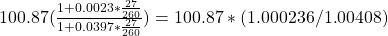Currency Exchange Rates | IFT World
IFT Notes for Level I CFA® Program
IFT Notes for Level I CFA® Program

# Part 4

## 6. Forward Calculations

Forward exchange rates are generally quoted in terms of points or pips. A spot rate is the rate that is in effect today. A forward rate is a fixed exchange rate that we lock in today based on which currencies will be exchanged at some future date.

The table below lists the spot rate and several forward rates for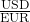currency pair. The negative sign indicates that the forward rate is less than the spot rate, i.e. the base currency EUR is weakening relative to the US dollar.

 Maturity Spot rate or forward points Spot 1.2875 One week – 0.3 One month – 1.1 Three months – 5.5 Six months – 13.3 Twelve months – 26.5

To convert forward points into a forward rate, divide the points by 10,000 if the exchange rate uses a four decimal place convention and by 100 if the exchange rate uses a two decimal place convention, and then add to the spot rate.

What is the 12-month forward rate?

Forward rate = 1.2875 + –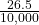= 1.28485

At times, forward points can also be expressed as a percentage of the spot rate. For example,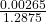* 100 = -0.21%

Relationship between Spot Rates, Forward Rates, and Interest Rates

The forward exchange rate depends on relative interest rates. To derive the relationship between spot rates, forward rates, and interest rates, assume you have one unit of domestic currency to be invested for let us say, one year. There are two options you may consider:

The exchange rate convention used =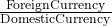=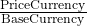Option 1Invest one unit of domestic currency (base currency) at the domestic risk-free rate iB for one period.

Amount at the end of the period = 1 + iB

Option 2: Convert one unit of domestic currency into foreign currency (base currency) today using the spot rate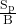. Invest this amount at the foreign risk-free rate  for one period. Determine the forward rate F_P/B today at which the price currency will be converted back to base currency.

Amount of units of price currency at the end of the period =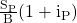Amount in terms of base (domestic currency) at the end of the period =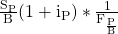Note: By using forward rate, any foreign exchange risk was eliminated in this transaction.

Since the risk of these two investments is the same, they should generate equivalent returns. As an investor, you should be indifferent between the two as it is a no-arbitrage relationship.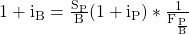The above equation can be rewritten as: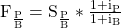where,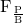= forward rate

iP = risk – free rate of price currency

iB = risk – free rate of base currency

Let us use some values now to compute the forward rate. The spot rate for INR/USD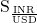= 100.

The risk-free rate for INR is 10% and the risk-free rate for dollar (base currency) is 1%.

Forward rate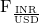=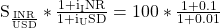= 108.9108

Some important points to be noted:

• The currency with the higher (lower) interest rate will always trade at a discount (premium) in the forward market.
• In our example above, USD was the base currency with a risk-free rate of 1%, while INR was the price currency with a risk-free rate of 10%. The forward rate calculated was 108.91 which means that the currency with the lower interest rate (USD) appreciated, while the currency with the higher interest rate (INR) depreciated. (Trading at a discount means the currency depreciates. Trading at a premium means the currency appreciates.)
• This relationship ensures that there is no arbitrage. The only forward rate that prevents arbitrage is 108.91. Otherwise, for any rate greater/lesser than 108.91 traders can exploit the mispricing by buying low and selling high.
• If the forward contract is for x days, make an adjustment based on the x/360 convention unless told otherwise. Refer to the equation below.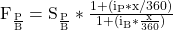Example

Given the following data, calculate the 37-day forward rate for JPY/CAD:

Canadian dollar risk-free interest rate = 3.97%

Japanese yen risk-free interest rate = 0.23%

Spot rate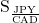= 100.87

Solution:

Forward rate =